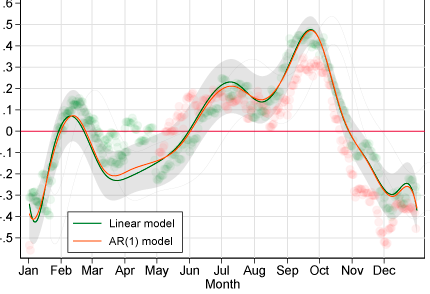# Analysis of Complex Sample Survey Data using StataDear all,

RE: Analysis of Complex Sample Survey Data using Stata

FineResults Research Services would like to invite you to high impact training on Analysis of Complex Sample Survey Data using Stata to be held in Nairobi from 13th-17th April 2020 .

COURSE PROFILE

Date: 13th-17th April 2020 .

Duration: 5 Days

Venue: FineResults Research Training Centre, Nairobi , Kenya

Cost: USD 800

Online Registration : REGISTER HERE

INTRODUCTION

Standard courses on statistical analysis assume that survey data arise from a simple random sample of the target population. Little attention is given to characteristics often associated with survey data, including missing data, unequal probabilities of selection, stratified multistage sample designs, and measurement errors. Most standard statistical procedures in software packages commonly used for data analysis do not allow the analyst to take these properties of survey data into account unless specialized survey procedures are used. Failure to do so can have an important impact on the results of all types of analyses, ranging from simple descriptive statistics to estimates of parameters of multivariate models. This course provides an introduction to specialized software procedures that have been developed for the analysis of complex sample survey data including testing for between-group differences in means and proportions, regression analysis, logistic regression and multilevel modeling. We will also consider the consequences of non response and missing data on survey analysis and methods for dealing with missing data. Specialized procedures for survey data analysis from the Stata systems for data management and analysis will be used to develop course examples and exercises

LEARNING OBJECTIVES

At the end of the training, participant will understand:

• Descriptive and inferential statistics

• Various data collection techniques and data processing methods e.g. Open data Kit (ODK)

• How to create and manipulate graphs and figures in STATA

• How to use STATA effectively for manipulating and analysing data.

DURATION

5 Days

COURSE OUTLINE

Module 1:

Introduction to Statistics, Data Collection, Processing and STATA

• Introduction to Statistics Concepts

• Data Processing and Management with STATA

• Concepts and Software and technologies for Data Collection and Processing

• Data Processing and Management with STATA

STATA Basics

• Data Structures and Types of Variables

• Data Management using STATA

• Output management and tabulating data with STATA

• Output Management

• Basics of STATA programming

• Tabulating data with STATA

Module 2:

Survey estimation and inference for complex designs

• Complex sample designs, survey estimation and inference

• Multi-stage designs, stratification, cluster sampling, weighting, item missing data, finite population corrections

• Models and assumptions for inference from complex sample survey data

• Sampling distributions, confidence intervals

• Design effects.

Module 3:

Basic STATA Graphics

• Basics of graphing

• Graphing quantitative

• Graphing qualitative data

Statistical Inference

• Tests of Association

• Tests of Difference

• Hypothesis testing

Regression Analysis using STATA

• Linear Regression

• Multiple Regressions

• Binary regression

• Logistic Regression

• GLM Model

• The Problems with regression

Module 4:

Advanced analysis of complex survey data

• Introduction to survey data

• Bayesian Analysis of Complex Sample Survey Data

• Generalized Linear Mixed Models (GLMMs) in Survey Data Analysis

• Fitting Structural Equation Models to Complex Sample Survey Data

• Small Area Estimation and Complex Sample Survey Data

• Nonparametric Methods for Complex Sample Survey Data

Panel data analysis

• Introduction to panel data analysis

Module 5:

Time series Analysis

• Basic elements of time-series analysis

• Managing and summarizing time-series data

• Univariate models

• Introduction to forecasting in STATA

NB: We are offering you a half day, fun and interactive team building event!

ACCOMMODATION

Accommodation is arranged upon request. For reservations contact us through Mobile: +254 732 776 700 / +254 759 285 295 or Email: [email protected]

PAYMENT

Payment should be transferred to FineResults Research Services Limited bank before commencement of training. Send proof of payment through the email: [email protected]

How to participate

Individual Registration

Contact information

Email: [email protected]

TEL: +254 732 776 700 / +254 759 285 295